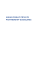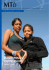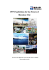# Testing Purchasing Power Parity in the Long-Run

## Transcription

Testing Purchasing Power Parity in the Long-Run
```Testing Purchasing Power Parity in the Long-Run
Ilona Shiller
University of New Brunswick
This paper studies the validity of the long-run purchasing parity hypothesis. The five currencies of
interest in this study are the U.S. dollar, the U.K. pound sterling, the German mark, the French franc, and
the Japanese yen. I attempt two approaches to test the long-run PPP hypothesis. First, I test for unit roots
of the real exchange rates themselves. Second, I examine the cointegration relationship between the
foreign dollar price level and the Canadian price level. Results show that the long-run PPP hypothesis
cannot be confirmed.
INTRODUCTION
The purchasing power parity (PPP) hypothesis is one of the most controversial topics in the field of
international finance. Many theoretical and empirical models have been built on the assumption that it
holds. Although, this topic has been researched extensively, no unique answer exists as to whether it
holds in the real life to date. It is a well-known fact that substantial departures from the PPP are possible
in the short-run. The empirical results on the long-run validity of the PPP have yielded contradictory
results depending upon the particular currencies under consideration, the price indices used to measure
price levels or inflation, the particular time period under investigation, and the method of the analysis
used. For example, a number of researchers such as Enders (1995), McDonald (1992), Zhou (1997), Chen
(1995) have demonstrated that the long-run PPP holds. Other researchers such as Cochran and DeFina
(1995), Fung and Lo (1992), In and Sugema (1995) have shown that departures from the PPP are
permanent. However, researchers such as Henricsson and Lundback (1995), Whitt (1989), and Glen
(1992) have obtained mixed results that were neither in favour nor against the PPP hypothesis.
In this study I use the time series of exchange rates and consumer price indices from January 1982 to
May 1997. The data set is downloaded from the following website: http://www.economagic.com/
Unfortunately, the data is discontinued and an analysis on an extended dataset is not possible. The five
currencies of interest in this study are the U.S. dollar, the U.K. pound sterling, the German mark, the
French franc, and the Japanese yen. Canada is used as the base country.
This study employs the univariate unit root approach to test for the stationarity of real exchange rates
of five industrialized countries. I also apply the Sims test, introduced by Christopher A. Sims in 1988, to
real exchange rates (Whitt, 1989). The Sims test is less sensitive to the spurious acceptance of the unit
root. An alternative way to check the validity of the PPP hypothesis is to conduct the cointegration
analysis of the foreign and local price levels. The Engle-Granger cointegration test shows that price levels
in the U.K. and Canada are cointegrated. I estimate the error-correction model for the U.K. and Canadian
price levels.
The rest of the paper is organized as follows. In the next section I give an overview of the PPP
concept. The third section presents a review of empirical problems that are associated with testing the
Journal of Applied Business and Economics vol. 14(1) 2013
11
PPP. The fourth section outlines the methodology used in this paper and presents empirical results. The
fifth section contains the conclusion.
THE CONCEPT OF PURCHASING POWER PARITY
The theory of the PPP is based on the law of one price, which says that prices of identical goods
should be equalized across markets. Under the conditions that the same goods are included in the price
indices and that price indices are constructed identically, the law of one price generalizes to the absolute
version of the PPP, which is given in the following equation:
Pd  P f  E ,
(1)
where E is the nominal exchange rate in period t; Pd is the domestic price level; and Pf is the foreign price
level.
An alternative way of expressing the absolute PPP is through the real exchange rate. The real
exchange rate is viewed as a measure of a country’s competitiveness versus another country (in our case,
versus Canada) and may be expressed as:
Rt  E   P f / P d 


(2)
If foreign prices are greater than domestic prices and this is not offset by a depreciation of the foreign
currency, then a real appreciation of the foreign currency will follow and, therefore, the foreign country
will become uncompetitive (Cooper, 1994). If the PPP holds so that E   P d / P f  , then the value of Rt


should be unity. Alternatively, taking logs of both sides of the equation (2) one gets the following
expression:
log Rt  log E  log P f  log P d
(3)
And because Rt should be unity in the equation (2), log Rt should be zero.
From the equation (3), the long-run PPP implies that if the nominal exchange rate, domestic and
foreign price indices are I (1), then they are cointegrated with the cointegration vector (1, -1, 1). Thus, the
long-run PPP can be validated if a cointegration vector, (1, -1, 1), can be found for the nominal exchange
rate and the two price indices. If the long-run PPP holds, then deviations from the PPP in the short-run are
assumed to be transitory. Since the real exchange rate measures the deviation from the PPP, testing the
PPP in the long-run is equivalent to testing the real exchange rate for stationarity.
A weaker version of the PPP, the relative PPP, states that the equation E   P d / P f  is expected to


hold up to a constant or that the nominal exchange rate moves to compensate for the differences in the
inflation rate:
E  Pd  P f
(4)
It has been argued in the literature that deviations from the PPP may be caused by a multitude of
different factors, including transportation costs, tariffs and other restrictions on trade, tax differences
across countries, the existence of non-traded goods and services, relative price changes, differential
speeds of adjustment in the currency exchange and goods markets, as well as problems associated with
the construction of price indices.
12
Journal of Applied Business and Economics vol. 14(1) 2013
A REVIEW OF EMPIRICAL PROBLEMS ASSOCIATED WITH TESTING PPP
The empirical studies of the PPP hypothesis often include a few common problems. I discuss some of
these problems next.
Choice of the Price Index
The choice is often made between the consumer price index (CPI), the wholesale price index (WPI),
and/or the GDP deflator. The CPI represents the consumer basket of goods, whereas the WPI represents a
basket of goods traded more often across nations. It is argued that the WPI is a more suitable choice for
the price index, because the inclusion of non-traded goods in price indices is often considered as an
explanation for deviations from the PPP. As Macdonald (1995) notes: “since both of these measures
incorporate prices of non-traded goods, it is unlikely that their use in an empirical test would produce the
symmetry and proportionality…, although these conditions are most likely to hold for tests construed
using wholesale prices, a series that contains a relatively large traded goods element.” Although, In and
Sugema (1995) argue that the definition of traded and non-traded goods vary widely across the countries.
The GDP deflator is viewed to be a superior measure of a country’s price level to either the CPI or the
WPI, because it assigns an appropriate weight to each good, no matter what classification is chosen.
Non-Stationarity of the Time-Series
The univariate tests frequently used to check for the stationarity of the series include: Dickey-Fuller
tests, augmented Dickey-Fuller tests, and Phillips-Perron tests. The Phillips-Perron tests allow
disturbances to be serially correlated as well as heteroscedastic. Therefore, Phillips-Perron tests are
deemed superior to Dickey-Fuller tests. The problem with the unit root tests is that they are biased toward
the acceptance of the unit root hypothesis unless there is strong evidence against it. The result also
depends on whether the constant and/or trend are included. If the model is incorrectly specified, the power
of the test decreases. Therefore, in practice one should estimate models with and without constant and/or
trend.
Sims, in 1988, proposed another test to test for a random walk. First, he suggests estimating the
following simple regression:
Yt  Yt 1   t
(5)
The value of the autoregressive  is crucial in evaluating the long-run behaviour of the real exchange
rate. This test is based on the “Bayesian posterior odds ratio” (Whitt, 1989). To apply the Sims test, one
needs to estimate the regression model (5), where the null hypothesis is that =1 and the alternative
hypothesis is that <1. In order to perform this test one needs to specify a prior distribution of . The
Sims approach puts a prior on the , which spreads the probability  of observing  evenly between 0 and
1. Sims suggests giving the unit root a probability of (1-), where  lies between 0 and 1. Sims thought
that unit roots are uncommon in economic data, and, therefore, suggested to use =0.8. This implies that
the unit root probability equals to 0.2. The test criterion is: The null hypothesis of a unit root is accepted if
Z>0, where Z=2*log ((1-)/)-log (p2)+2*log (1-2-1/s)-2*log {()}-log (2)-2, and  is the estimate of
 obtained from the regression of a real exchange rate on its own lagged value; p is the standard error of
;  is the conventional t-statistic for testing that =1 and equals to (1-)/p; () is the cumulative
distribution function for the standard normal distribution evaluated at ; and s is the number of periods per
year (s=12 for monthly data). The alternative hypothesis is accepted if Z is less than 0.
If nominal exchange rates and price series are I (1), then there exist an alternative way to check the
theory of PPP. This method is called the cointegration technique. The most widely used method to check
for cointegration is the Engle-Granger method. The advantage of the Engle-Granger approach is that
coefficient estimates of the cointegrating regression are super consistent in that they approach their
asymptotic values at the rate equal to T rather than the conventional T1/2. If there is a possibility for more
Journal of Applied Business and Economics vol. 14(1) 2013
13
than one cointegrating vector, the Johansen procedure is deemed to be more appropriate. The Johansen
procedure allows one to test for the presence of multiple cointegrating vectors. However, Cheung and Lai
(1993) indicate that asymptotic critical values of Johansen’s likelihood ratio tests can be biased towards
finding cointegration in finite samples. Huang and Yang (1996) use the Monte-Carlo simulation and find
that the Johansen procedure has a bias towards supporting the existence of cointegration, especially in the
case when the assumption of normally and identically distributed error terms is violated. They, as well as
Cheung and Lai (1993), claim that the Engle-Granger two-step method has greater power than the
Johansen maximum likelihood approach for testing the existence of cointegration if even one of the
variables entering the system deviates from normality.
Problems of Simultaneity
It is possible in the context of testing the PPP that both exchange rates and prices may be endogenous
(Mcdonald, 1995). For example, some academicians argue that the PPP can be falsely rejected when it is
true, because the estimates of price coefficients will be biased towards zero. The problem can be removed
using the instrumental variable method of estimation. Sharma, Mathur, and Wong (1991) perform
Granger causality tests and conclude that their results do not strictly support the view that prices cause
exchange rates. It should be said that the problem of simultaneity bias does not arise in the cointegration
framework of Engle and Granger. If prices and exchange rates are integrated of the same order and
cointegrated, the tendency of the low-frequency components to dominate the high-frequency components
in the cointegration regression will allow the researcher to abandon an explicit consideration of the
“direction of causality.” (Anonymous, 1992).
Cross-Sectional and Panel Data Estimation of PPP
Some researchers argue that in situations where the time series variation in the data is insufficient in
order to get reasonably good power for the unit root testing, combining data into cross-sectional data or
pooling can result in a substantial improvement. Panel unit root tests involve estimation of the following
k
regression: R jt   j  R jt 1   c ji R jt 1   jt , where the subscript j=1… n indexes the countries.
i 1
The panel test is designed to evaluate the null hypothesis that each individual series are I (1) versus an
alternative hypothesis that all series in a panel are stationary. This method was popularized by Levin-Lin
and applied by MacDonald (1995). This testing method produces a single t-ratio for the panel and this
statistic is demonstrated to have a standard normal distribution. The results of MacDonald’s (1995)
research indicate that the null of the unit root for his sample of real exchange rates is rejected, whereas
that of the stationarity - accepted.
THE EMPIRICAL RESULTS
The long-run PPP is said to hold if constructed series of real exchange rates are stationary. A critical
first step to determine whether real exchange rates are, indeed, stationary is to visually inspect the data.
The trajectories of all 5 real exchange rate series are illustrated in FIGURE 1 below.
14
Journal of Applied Business and Economics vol. 14(1) 2013
FIGURE 1
THE TRAJECTORIES OF REAL EXCHANGE RATES
Year
UK
Germany
France
US
Yen
Recall, that the PPP hypothesis holds if the logarithm of the real exchange rate equals to zero. As can
be seen from FIGURE 1, real exchange rates are not exactly equal to zero, but rather exhibit the random
walk behaviour. Autocorrelation functions of real exchange rates in the level form, which are not reported
here to save space, show almost no tendency to decrease, whereas those in the differenced form show a
pattern similar to that observed for stationary time-series.
I also perform a graphical analysis of the absolute PPP over time for selected countries. I compare
spot exchange rates (relative to the Canadian dollar) with relative prices (Pd/PC\$). An overvalued currency
is one for which the ratio of price indices exceeds the exchange rate. I demonstrate that departures from
the PPP are common. For example, the U.S. dollar, and the U.K. pound sterling have been consistently
overvalued, whereas the Japanese yen and the French franc have been consistently undervalued against
the Canadian dollar. Moreover, it is not obvious that there is a tendency for deviations to disappear over
time. For the German mark, however, the spot exchange rate tracks relative prices reasonably well.
TABLE 1 gives the summary statistics on considered real exchange rates. Using Canada as the base
country, series are calculated for five other industrialized nations: the U.K., the U.S., France, Germany,
and Japan. The span of the time covered belongs to the period of flexible exchange rates, and the data
have been normalized to make the value of real exchange rates equal to zero in January 1982. Because the
data is expressed in logarithms, the mean represents the average discrepancy in percent over the entire
period between the real exchange rate and its value in January 1982. For example, the mean for the
United States is –5.9%, implying that the Canadian dollar was, on average, 5.9% less valuable relative to
the U.S. dollar than it had been in January 1982.
The second column of the table gives the standard deviation, which is a measure of the dispersion
around the mean. This indicator shows that real exchange rates have exhibited some variation. For
example, a standard deviation of 8.98 percent for the Japanese real exchange rate implies that roughly
one-third of the time this rate is more than 8.98 percent away from its average value.
The last two columns of TABLE 1 report the maximum and minimum values reached by each
exchange rate during the period covered. In all five cases the switch from high to low was at least 50
percent, thus reinforcing the conclusion that exchange rates fluctuate considerably. In contrast, if the PPP
hypothesis held exactly each month, real exchange rates would have been constant.
Journal of Applied Business and Economics vol. 14(1) 2013
15
TABLE 1
SUMMARY STATISTICS FOR REAL EXCHANGE RATES
Country
Mean
Percentage
Variation*
(Jan-82 = 100)
United States
United Kingdom
Japan
France
Germany
-5.9
4.9
-26.2
1.1
-17.2
Standard
Deviation
(percent)
Maximum
Minimum
2.4
5.9
8.98
2.6
6.9
0.18
15
-5.2
6.4
-0.65
-9.7
-3.3
-38
-3.4
-27.6
I use three different models to test real exchange rates for stationarity. The first is a pure random walk
model, the second includes an intercept or drift term, and the third includes both a drift and a linear time
trend. The problem is that in order to estimate these models, one should choose the appropriate lag length.
Including too many lags can reduce the power of the test to reject the null hypothesis of a unit root since
additional parameters are added to the equation, which constitutes a loss of degrees of freedom. However,
including too few lags will lead to the imprecise estimation of  and its standard error. I use the BoxJenkins methodology to select the appropriate lag length. I use the pure random walk model with the
initial lag length set at 12. It is important to allow for the lag length no shorter than 12 month with
monthly data. If the t-statistic on lag 12 is insignificant at 5% critical level, I re-estimate the regression
using a length of lag of 12-1. Such a procedure is repeated until I find a lag that is significantly different
from zero. It is often claimed by econometricians that this procedure in the autoregressive case will yield
the true lag length. Also Dickey-Fuller F-tests are performed in order to determine the appropriate lag
length. Phillips-Perron test statistics are also obtained and compared with their critical values. Results of
univariate tests1 (Dickey-Fuller, augmented Dickey-Fuller tests, and Phillips-Perron tests) are
predominantly favouring the unit root hypothesis of real exchange rates for selected countries.
For robustness purposes, I perform the Sims test. To do this, the log of the real exchange rate is
regressed on its own lagged value to get estimates of  and . The resulting estimates of the
autoregressive coefficient  are substantially close to 1. Then, -statistics are computed according to the
formula:  = (1-)/p. Because calculated  values for all countries are greater than the critical value of –
2.89, I cannot reject the unit root hypothesis on the basis of the conventional t-test.
Another statistic for testing the Sims method includes the Z-statistic. The Sims test accepts the null
hypothesis of a unit root if the calculated Z-statistic is positive. The middle column of the TABLE 2
reports Z values. These values are calculated using the prior probability for a unit root of (1-)=0.2. In all
five instances, the calculated Z values are negative, favouring the alternative hypothesis that considered
real exchange rates are stationary.
Whitt (1989) notes that a measure of the strength of rejection of the null hypothesis can also be
calculated. Having information with respect to the estimates of  and , I can “calculate the smallest
probability on the null hypothesis, (1-*), that would be necessary in order to force the Sims criterion to
favour the random-walk hypothesis”(Whitt, 1989). So, whether the rejection of the null hypothesis is
strong or weak can be deduced from the value of the (1-*): the greater the value of the (1-*), the
stronger the rejection of the null of a unit root. As demonstrated in the third column of the table 2, the null
is rejected fairly strongly.
16
Journal of Applied Business and Economics vol. 14(1) 2013
TABLE 2
SIMS TEST FOR A UNIT ROOT OF THE REAL EXCHANGE RATE
Country
US
UK
France
Germany
Japan
Dickey-Fuller

0.35956
-1.26156
1.00944
-1.65662
-2.40704
Z
-1.41184
-2.375084
-2.282471
-2.477548
-4.2248
Sims test
(1-*)
0.328105
0.451001
0.441173
0.461479
0.593707
The null hypothesis for both tests is that =1. The critical value for the  is –2.89, whereas for the Sims test
the critical region is Z<0
The theory asserts that the logarithm of Rt should be a zero mean stationary process, or an integrated
of order one process. Results of our analysis are contradictory and indicate that real exchange rates are I
(1) according to standard univariate unit root tests and I (0) according to the Sims test. However, these
results cannot be contrasted. If univariate unit root results are, in fact, true, then I can assert that there is a
tendency for the nominal exchange rate and the price ratio to drift apart.
The theory of the PPP also does not allow for multiple unit roots. Therefore, first differences of real
exchange rates are checked for stationarity. Results of Dickey-Fuller tests show that first differences of
real exchange rates for Japan, France, and Germany are nonstationary. Due to the latter finding, I adopt a
Dickey and Pantula strategy of testing for multiple unit roots. Enders (1995) writes that if this strategy
yields no evidence of multiple unit roots, one can conclude that the time-series are stationary. I apply this
methodology and find no multiple unit roots for real exchange rates applicable to Japan, France, and
Germany.
THE COINTEGRATION ANALYSIS
Recall, E, Pd, and Pf represent the logarithms of the price of foreign exchange, foreign price level, and
domestic price level respectively. The long-run PPP states that real exchange rates, defined as E= Pf- Pd,
should be stationary. The unit root tests indicate that these series are non-stationary. Cointegration is an
alternative way to check the theory. If the PPP holds then I should expect that the series {E*Pf}, which is
the dollar value of the foreign price level Ft, will be cointegrated with the Canadian price level, Pd.
Testing foreign price levels and Canadian price levels in the level and first differenced form indicate that
all of these series are I (1)2. Autocorrelation functions of price level series in the level form resemble
those of non-stationary unit root processes, except for the dollar German price. Standard univariate unit
root methods also show that the dollar German price is a stationary process.
In making a choice between the Engle-Granger approach for testing cointegration and the Johansen
procedure, one needs to be certain about the distributional properties of error terms. Based on numerous
Monte Carlo experiments, researchers agree that the power of the Engle-Granger two-step method is
greater than that of the Johansen maximum likelihood approach for testing the existence of cointegration
if one of the variables entering the model is not normally distributed. The normality of disturbance terms
is tested. Specifically, the Jargue-Bera (J-B) test of normality, as well as the Box-Pierce Q test is
performed. Results of these tests show that all of the first differenced variables are not normally
distributed. Due to the latter fact, I chose the Engle and Granger approach for testing cointegration.
The long-run PPP, if holds, states that residuals formed from the equilibrium regression3 are
stationary and that the cointegrating vector is =1. The results of equilibrium regressions are given in
TABLE 3.
Journal of Applied Business and Economics vol. 14(1) 2013
17
TABLE 3
THE EQUILIBRIUM REGRESSIONS
Country
Coeffi
cient
Value
Stand. Error
t-value
R2
DW
US

-2.81597
0.66396
-5.54772
1.86093
2.45198
0.50758
3.07491
0.63437
0.47095
0.09716
0.03584
0.00739
-0.91600
1.047
-11.78000
19.153
68.414
68.647
0.005951
0.02963
344.9995
Deg.
Freed
om
183
0.667184
0.1116
80.92797
183
0.96262
0.13283
4.69E-02
183
-0.75924
1.28822
0.10831
0.02235
-7.01
57.649
0.9478
0.0661
0.428082
183


UK

Japan


France


The residuals obtained from each regression equation, called t, were checked for unit roots. Since the
residuals from a regression equation have a zero mean and do not have a time trend, the following two
equations are applied to residuals from each equilibrium relationship4:
 t   1  t 1   1t
(6)
 t   1  t 1    i 1  t  i   2 t
(7)
If 1 equals to 0, then the null of no cointegration cannot be rejected. The Dickey-Fuller statistic
tables are, however, inappropriate here because the residuals used in (6) and (7) are not the actual error
terms, but the estimated error terms obtained from running the equilibrium regression. Then I compare the
estimated t-values for 1 from regressions (6) and (7) with the calculated critical values of Engle and
Granger5. The results of testing residuals from the equilibrium regressions for stationarity are given in
TABLE 4.
TABLE 4
TESTING THE RESIDUALS FROM THE EQUILIBRIUM REGRESSIONS FOR
STATIONARITY
Country
US
UK
Japan
France
Estimated
regression
Value of
1
Standard
Error
tstatistics
BG
test
compared to
critical value
Order
of
autocorrelation
PhillipsPerron 
test
PhillipsPerron t
test
Regression
(1)
Regression
(2)
Regression
(1)
Regression
(2)
Regression
(1)
Regression
(2)
Regression
(1)
Regression
(2)
-0.0144
0.01272
-1.1310
31.76>18.3
10
-3.845
-1.37
-0.0157
0.0127
-1.249
-0.0679
0.02421
-2.808*
44.28>7.81
3
-13.65
-2.90*
-
0.02427
-3.872*
-0.0716
0.02648
-2.705*
76.34>21.03
12
12.87
0.23
-0.0369
0.02558
-1.442
-0.0371
0.01881
-1.933
15.17>7.815
3
-8.015
-2.11
-0.0483
0.01940
-2.489
0.09398
*indicates significance at the 5% critical value
18
Journal of Applied Business and Economics vol. 14(1) 2013
Since in absolute terms the estimated -values for both regressions in an application to U.K. exceeds
any of the critical values at the 5% significance level, the conclusion is that the estimated residuals are
stationary, and, therefore, the U.K. dollar price level and the Canadian price level are cointegrated.
Moreover, calculated Phillips-Perron t-test is also statistically significant confirming that the abovementioned variables are cointegrated. Thus, it is possible to reject the null hypothesis of no cointegration
only for the U.K. For all other countries the null hypothesis of no cointegration cannot be rejected and,
therefore, it can be concluded that the PPP has generally failed.
Finally, I estimate the U.K./Canadian error-correction model. The lag length is chosen based on
results of F-tests and cross-equation likelihood ratio tests. The final versions of error correction models
for the U.K. and Canadian price levels during the period of January 1982 to May 1997 are:
Ft  0.003715 (0.005053 )  0.062187 (0.005053)  t -1   3t
(8)
Pt  0.002977 (0.000257 )  0.003128 (0.001226 )  t 1   4 t
(9)
where t-1 is the lagged residual from the equilibrium regression of the U.K. price level on the Canadian
price level; standard errors are given in the brackets. Taking a closer look at the results of the error
correction models, one can see that the point estimates in (8) and (9) show a direct convergence towards
the long-run equilibrium. For example, when there is a one-unit deviation from the long-run PPP in time
period t-1, the U.K. price level falls by 0.062187 units and the Canadian price level rises by 0.003128
units. Both these price changes will work to eliminate any divergence from the long-run PPP in time
period t-1. Notice that the speeds of adjustment terms are significantly different from zero for both
Canada and the U.K. (the error-correction term is about 2.578 deviations from zero for the U.K. and 2.551
deviations from zero for Canada). This result is consistent with the idea that both countries are large and,
therefore, have an impact on movements of the respective exchange rate.
CONCLUSION
In this study, using the time series of exchange rates and consumer price indices, I test the validity of
the long-run PPP hypothesis. The five currencies of interest are the U.S. dollar, U.K. pound sterling,
German mark, French franc, and the Japanese yen. I attempt two approaches: (1) testing for unit roots of
real exchange rates themselves; and, (2) testing the cointegration relationship between the foreign dollar
price level and the Canadian price level.
Results of the Sims test suggest that real exchange rates are stationary, lending validity to the PPP
hypothesis. In contrary, results of univariate unit root tests show that the PPP has generally failed.
Although, alternative tests for unit roots may be more successful in rejecting the null hypothesis of the
unit root such as variance ratio tests, MacDonald (1995) asserts that “the degree of mean reversion”
remains to be “painfully slow.” The cointegration hypothesis between the foreign and Canadian price
levels is confirmed for the U.K. only.
ENDNOTES
1.
2.
3.
4.
Results of the unit root testing are available upon request.
Results are available upon request.
The Engle and Granger equilibrium regression takes the following form: Ft =  +  Pt + t
In applied econometrics, equation (6) is used only if residuals from the equilibrium regressions are
serially uncorrelated. Because I have doubts with regard to whether the errors are white noise, I am
also using the augmented form of the test, represented by the regression (7). The unaugmented tests
have limited power if residuals are serially correlated.
5. Critical values for cointegration tests are computed from the equation given in J. G. MacKinnon with
T=184, “Critical values for cointegration tests,” Cointegrated Time Series: 267-276. For the
Journal of Applied Business and Economics vol. 14(1) 2013
19
regression model with no lags, the 99%, 95%, and 90% confidence levels correspond to critical
values of -2.58, -1.94, and -1.62 respectively. For the regression model with lags, the 99%, 95%, and
90% confidence levels correspond to critical values of -3.73, -3.17, and -2.91 respectively.
REFERENCES
Chen, B. (1995). Long-run Purchasing Power Parity: Evidence from Some European Monetary System
Countries. Applied Economics, 27, 377-383.
Cheung, Y., & Lai, K. S. (1993). Finite Sample Size of Johansen’s Likelihood Ratio Test for
Cointegration. Oxford Bulletin of Economics and Statistics, 55, August, 313-328.
Cochran, S. J. and DeFina, R. H. (1995). Predictable Components in Exchange Rates. The Quarterly
Review of Economics and Finance, 35, Spring, 1-14.
Enders, W. (1995). Applied Econometric Time-Series. John Wiley & Sons, Inc.
Fung, H. and Lo, W. (1992). Deviations from Purchasing Power Parity. The Financial Review, 27,
November, 553-570.
Glen, J. D. (1992). Real Exchange Rates in the Short, Medium and Long-run. Journal of International
Economics, 33, 147-166.
Henricsson, R & Lundback, E. (1995). Testing the Presence and the Absence of Purchasing Power Parity:
Results for Fixed and Flexible Regimes. Applied Economics, 27, 635-641.
Huang, B. & Yang, C. W. (1996). Long-run Purchasing Power Parity Revisited: a Monte-Carlo
Simulation. Applied Economics, 28, 967-974.
In, F. & Sugema, I. (1995). Testing Purchasing Power Parity in a Multivariate Cointegrating Framework.
Applied Economics, 27, 891-899.
MacDonald, R. (1995). Long run Exchange Rate Modelling: A Survey of Recent Evidence. IMF Staff
Papers, 42, 437-483.
MacDonald, R. (1992). Long run Purchasing Power Parity: Is it for Real? The Review of Economics and
Statistics, 3, 690-695.
Sharma, S. C., Mathur, I. & Wong, J. P. H. (1991). Causality in Purchasing Power Parities. Quarterly
Review of Economics and Business, 31, Spring, 81-89.
Whitt, J. A. (1989). Purchasing Power Parity and Exchange Rates in the Long-run. Federal Reserve Bank
of Atlanta Economic Review, 74, 18-32.
Zhou, S. (1997). Purchasing Power Parity in high-Inflation Countries: A Cointegration Analysis of
Integrated Variables with Trend Breaks. Southern Economic Journal, 64, 450-467.
20
Journal of Applied Business and Economics vol. 14(1) 2013
```

### Infrastructure Investment in Mexico: Challenges and Opportunities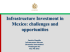### Britco Supplies Disaster Relief Housing for Save the Children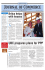### The Philippines: A New Awakening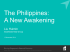### INTRODUCTION X-ray fluorescence spectrometry is one of the most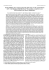### To Address the Key Challenges of Converting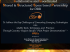### Playbill - Running To Places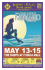### Playbill - Running To Places### File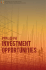### Presentation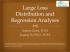### asean public private partnership guidelines